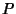## Percent

The use of percentages is a way of expressing Ratios in terms of whole numbers. Given a Ratio or Fraction, it is converted to a percentage by multiplying by 100 and appending a ``percentage sign'' %. For example, if an investment grows from a numberto a number, thenistimes as much as, or 173.08%, and the investment has grown by 73.08%.